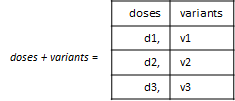Combine Simulation Scenarios in SimBiology

There are two different methods to combine SimBiology simulation scenarios that have different doses, different variants, or different sample values for model quantities. After the combination, you get a final set of values (parameter set) that you can use to simulate and explore the model behavior. For instance, you can combine different dosing regimens with different categories of patients (variants) and simulate to explore the efficacy of the drug.

Cartesian Combination

This method is the Cartesian product of two sets. Suppose doses is a set of different RepeaDose objects [d1,d2,d3], and variants is a set of variant objects: [v1,v2,v3]. The Cartesian combination of doses and variants is: doses x variants = (d1,v1),(d2,v1),(d3,v1),(d1,v2),(d2,v2),(d3,v2),(d1,v3),(d2,v3),(d3,v3).Elementwise Combination

This combination method combines the entries one to one, that is, elementwise. In other words, the first element of the first set (entry) is combined with the first element of the second set (entry) and so on. Using the same variables above, the elementwise combination of doses and variants is: doses + variants = (d1,v1),(d2,v2),(d3,v3). Both sets must have the same number of elements (samples) for this combination method.